# EVERYTHING YOU NEED TO LEARN ABOUT LIST IN PYTHON PROGRAMMING

in STEMGeeks12 days ago

Python has four built-in data types which are: list, tuple, set and dictionary. So, lists in python are data structures that you can use for storing items in a particular order. For example: list can be used to store the names or number and we will see this in a while. List in python is represented by square brackets ([ ]) and each elements in the list are separated by commas. Lists can have different kind of items like integer value, string value, Boolean value or a combination of these. And other thing is that you can use list if you want to store duplicate values as well.

Lets learn a few cool concepts about the list. For this open your IDE. I am using PyCharm. I will create a new file and you can named it as you like. Lets create a simple list and print the list:

``````list1 = [12, 13, 45, 54, 23, 90, 101, 203, 40, 13, 34, 27, 76, 44]
print(list1)
``````

This will give output as below: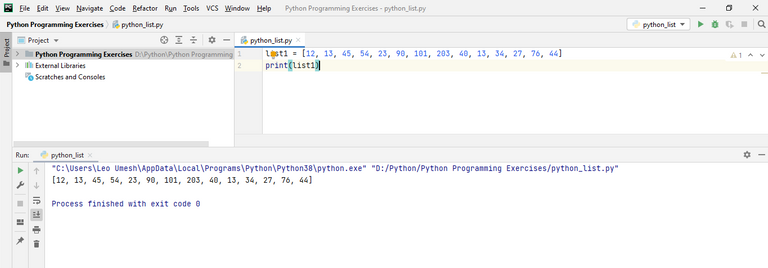You can see here 13 is being printed twice because list allows to store duplicate values as I mentioned earlier.

In order to access the individual element of the list, you can pass its index number. Just write down the list name along with square bracket and write down the index number or position in the bracket. Always remember the first element in the list always has index value of 0. It doesn't start with 1. In the above code, lets say we want to get 23 and its index value is 4. So we will do this by following:

``````print(list1)
``````

If you run your code now, you will get 23 in the output screen.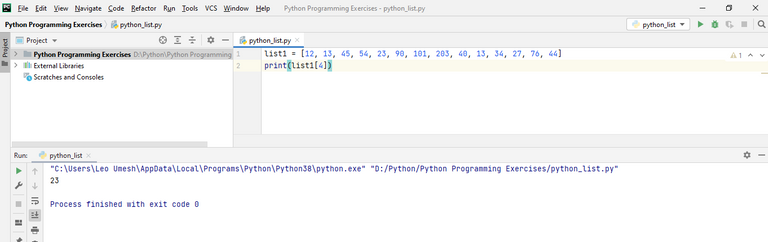In order to get the length of the list you can use `len()` method. For example to print the length of above list1, we will write `print(len(list1))`.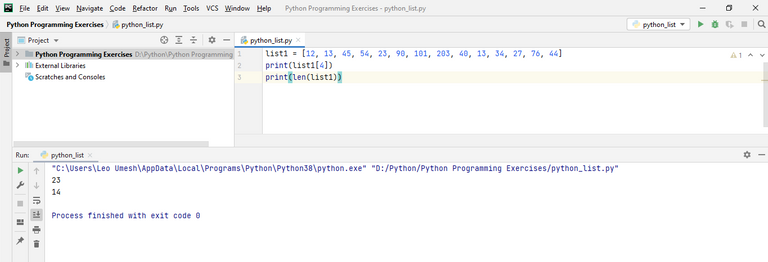Now lets see how to modify the values of list. Suppose we want to modify the value of the first element in the list in above code. For this we just specify the value for that index using "=" sign.

``````list1 = [12, 13, 45, 54, 23, 90, 101, 203, 40, 13, 34, 27, 76, 44]
print(list1)
list1 = 18
print(list1)
``````

We have printed our original list here and then changed the first element's value from 12 to 18 and then printed the same list again. You will get following output: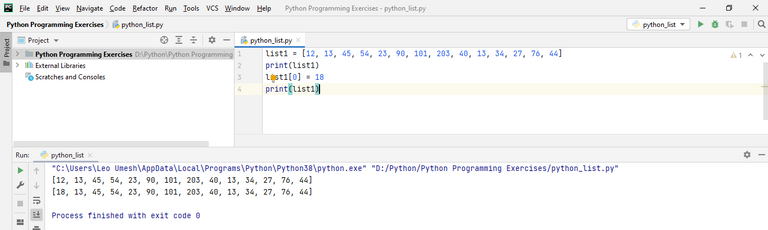Lets say we want to add a new value to the list in the above code. For this we use `append()` method. This will always add a new value to the end of the list. Lets see a simple example for now with the same above code.

``````list1 = [12, 13, 45, 54, 23, 90, 101, 203, 40, 13, 34, 27, 76, 44]
print(list1)
list1.append(53)
print(list1)
``````

The output is: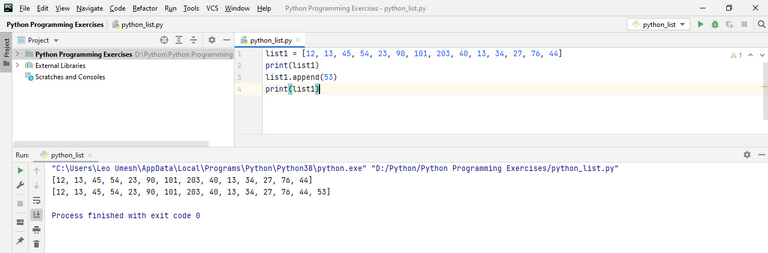The problem with this approach is that the new value always gets added to the end of the list. If you want to add a new value in your desired location or index, you can use `insert()` method. You will need to provide two parameters for this: one is the index number, where you want to insert the value and the other parameter is the value itself. Lets work with the above code:

``````list1 = [12, 13, 45, 54, 23, 90, 101, 203, 40, 13, 34, 27, 76, 44]
print(list1)
list1.insert(3, 53)
print(list1)
``````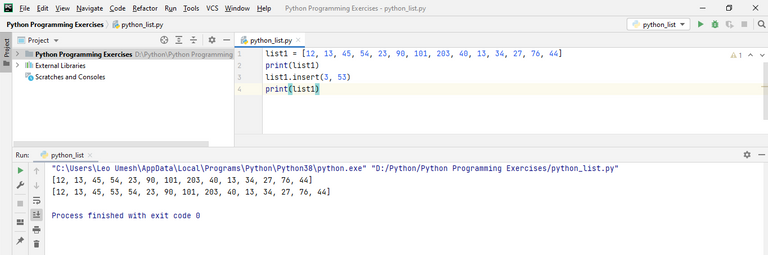You can see the new value gets inserted into the fourth position (As I told above, index number always starts from 0. So index number 3 means fourth value🙂).

Now we will see examples on removing items from the list. You can use two methods `del` and `pop()` for this. First now, lets see example of `del` keyword.

``````list1 = [12, 13, 45, 54, 23, 90, 101, 203, 40, 13, 34, 27, 76, 44]
print(list1)
del list1
print(list1)
``````

What this code will do is first print our original list and then delete the value in the second position as index number is specified as 1. Then it will print the list again after deleting. This is the output: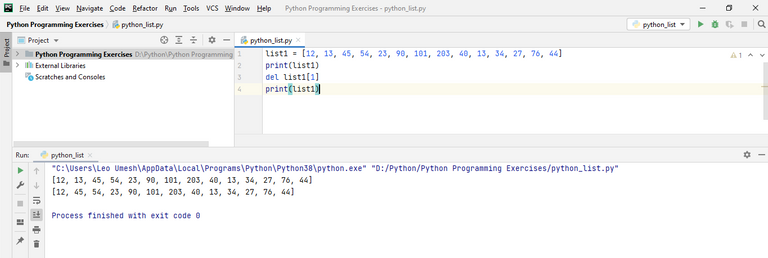You can use this `del` method for deleting the value in the position that you desired but using `pop()` will always delete the items in the list starting from the last one. For example, in the above code, we can use this same method to see what I told just now:

``````list1 = [12, 13, 45, 54, 23, 90, 101, 203, 40, 13, 34, 27, 76, 44]
print(list1)
list1.pop()
print(list1)

``````

The output will be: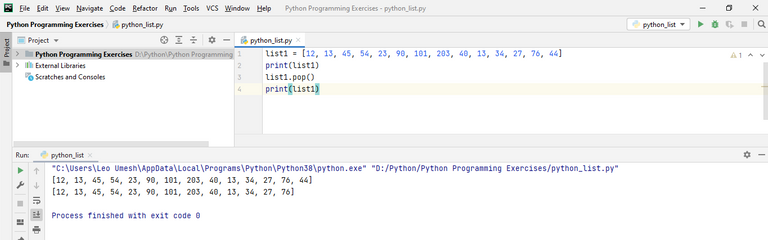However, you can also use `pop()` method to remove any desired items from the list. For example you want to pop the first element in the list so you should write `pop(0)`.

``````list1 = [12, 13, 45, 54, 23, 90, 101, 203, 40, 13, 34, 27, 76, 44]
print(list1)
list1.pop(0)
print(list1)
``````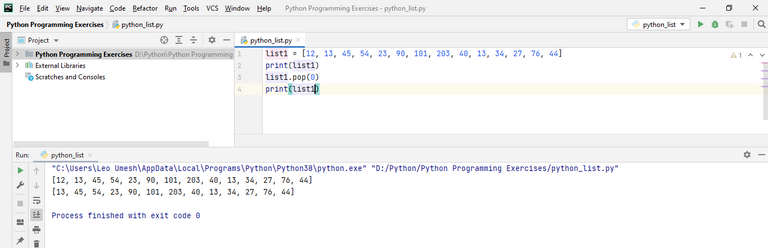Also another way to removing an item from the list is by specifying its value. For example if you want to delete the item from the above list with value 90, you should write `list1.remove(90)`. See this code for more:

``````list1 = [12, 13, 45, 54, 23, 90, 101, 203, 40, 13, 34, 27, 76, 44]
print(list1)
list1.remove(90)
print(list1)
``````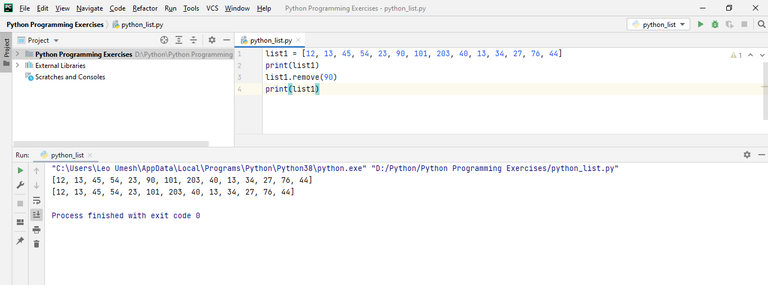Now lets learn how to sort the list. You can sort the value in the list whether its numerical or character or string values. I will show you sorting with both. I will create two lists, one list will contain numerical value and the other will contain character value.

``````list1 = [12, 13, 45, 54, 23, 90, 101, 203, 40, 13, 34, 27, 76, 44]
list1.sort()
print(list1)

list2 = ["Valerie", "John", "Anon", "Zoe", "Alice", "Ryan", "Donnie"]
list2.sort()
print(list2)
``````

If you execute the above code, this will be the output: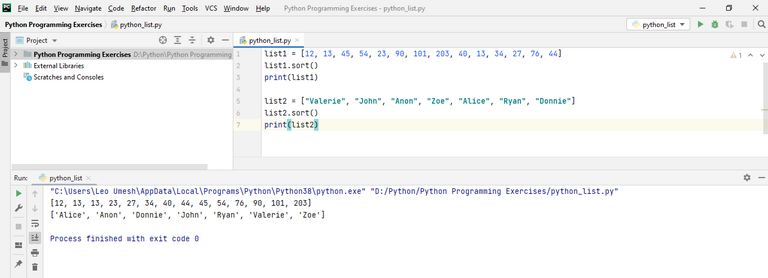If you want to reverse the list, you can use `reverse()` method. I will apply this to the above same code:

``````list1 = [12, 13, 45, 54, 23, 90, 101, 203, 40, 13, 34, 27, 76, 44]
list1.reverse()
print(list1)

list2 = ["Valerie", "John", "Anon", "Zoe", "Alice", "Ryan", "Donnie"]
list2.reverse()
print(list2)
``````

If you run this code, you can see your lists will be printed in the opposite order as below: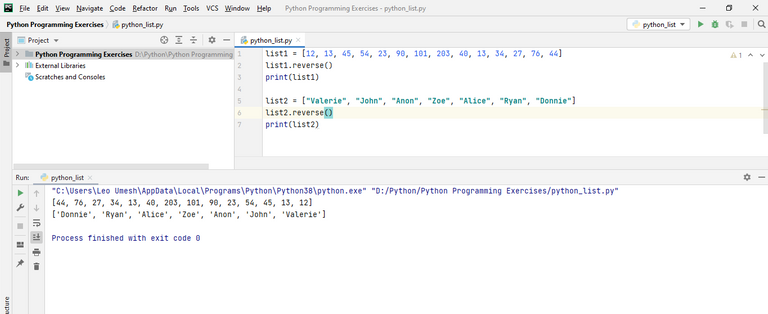The last thing I gonna do in this post is accessing each elements of list through looping and printing them. Its very simple to do this:

``````list1 = [12, 13, 45, 54, 23, 90, 101, 203, 40, 13, 34, 27, 76, 44]

for i in list1:
print(i)
``````

This will give you following output: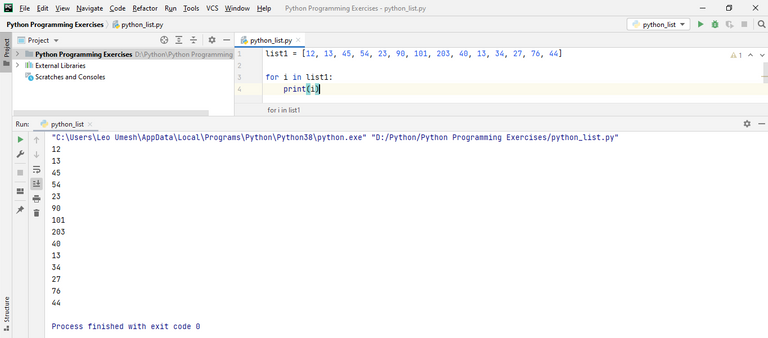Let me show another cool feature of looping through list. Suppose we had a list of names with lowercase letter. Then we will use for loop to print each name with its first letter capitalized. We will use in-built `title()` method for this one:

``````list2 = ["valerie", "john", "anon", "zoe", "alice", "ryan", "donnie", "brock"]

for i in list2:
print(i.title())
``````

You can see the first letter gets capitalized when you run this program.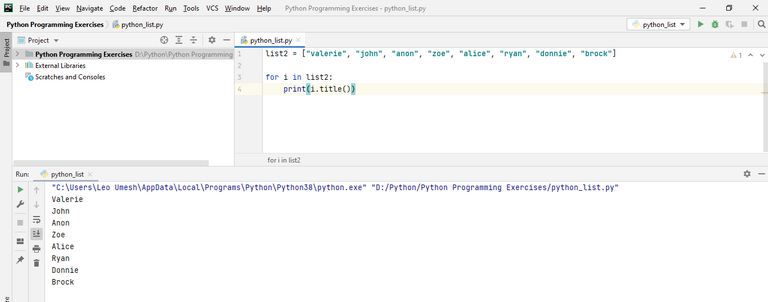There is more cool things you can do with the list but as this is getting long as of now, I will be writing these things in my upcoming blog posts. Thanks for reading my post for now.